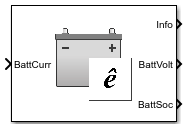Documentation

### This is machine translation

Mouseover text to see original. Click the button below to return to the English version of the page.

Note: This page has been translated by MathWorks. Click here to see
To view all translated materials including this page, select Country from the country navigator on the bottom of this page.

# Estimation Equivalent Circuit Battery

Resistor-capacitor (RC) circuit battery that creates lookup tables

• Library:
• Powertrain Blockset / Energy Storage and Auxiliary Drive / Network Battery## Description

The Estimation Equivalent Circuit Battery block implements a resistor-capacitor (RC) circuit battery model that you can use to create lookup tables for the Equivalent Circuit Battery block. The lookup tables are functions of the state-of-charge (SOC).

The Estimation Equivalent Circuit Battery block calculates the combined voltage of the network battery using parameter lookup tables. The tables are functions of the SOC. To acquire the SOC, the block integrates the charge and discharge currents.

Specifically, the block implements these parameters as lookup tables that are functions of the SOC:

• Series resistance, Ro=ƒ(SOC)

• Battery open-circuit voltage, Em=ƒ(SOC)

• Network resistance, Rn=ƒ(SOC)

• Network capacitance, Cn=ƒ(SOC)

To calculate the combined voltage of the battery network, the block uses these equations.

`$\begin{array}{l}{V}_{T}={E}_{m}-{I}_{batt}{R}_{o}-\sum _{1}^{n}{V}_{n}\\ {V}_{n}=\underset{0}{\overset{t}{\int }}\left[\frac{{I}_{batt}}{{C}_{n}}-\frac{{V}_{n}}{{R}_{n}{C}_{n}}\right]dt\\ SOC=\frac{-1}{{C}_{batt}}\underset{0}{\overset{t}{\int }}{I}_{batt}dt\\ {I}_{batt}={I}_{in}\\ {V}_{out}={V}_{T}\end{array}$`

Positive current indicates battery discharge. Negative current indicates battery charge.

The equations use these variables.

 SOC State-of-charge Em Battery open-circuit voltage Ibatt Per module battery current Iin Combined current flowing from the battery network Ro Series resistance n Number of RC pairs in series Vout, VT Combined voltage of the battery network Vn Voltage for `n`-th RC pair Rn Resistance for `n`-th RC pair Cn Capacitance for `n`-th RC pair Cbatt Battery capacity

## Ports

### Inputs

expand all

Combined current flowing from the battery network, Iin, in A.

### Output

expand all

Bus signal containing these block calculations.

SignalDescriptionVariableUnits

`CapVolt`

Voltage for `n`-th RC pair

VnV

Combined voltage of the battery network, Vout, in V.

Battery state-of-charge, SOC.

## Parameters

expand all

#### Core Battery

Number of series RC pairs. For lithium, typically 1 or 2.

Open-circuit voltage table, Em, in V. Function of SOC.

Series resistance table, Ro, in ohms. Function of SOC.

State-of-charge (SOC) breakpoints, dimensionless.

Battery capacity, Cbatt, in Ah.

Initial battery capacity, Cbatto, in Ah.

Initial capacitor voltage, in V. Dimension of vector must equal the Number of series RC pairs.

#### R and C Table Data

Network resistance table data for `n`-th RC pair, as a function of SOC, in ohms.

Network capacitance table data for `n`-th RC pair, as a function of SOC, in F.

#### Cell Limits

Upper voltage limit, in V.

Lower voltage limit, in V.

 Ahmed, R., J. Gazzarri, R. Jackey, S. Onori, S. Habibi, et al. "Model-Based Parameter Identification of Healthy and Aged Li-ion Batteries for Electric Vehicle Applications." SAE International Journal of Alternative Powertrains. doi:10.4271/2015-01-0252, 4(2):2015.

 Gazzarri, J., N. Shrivastava, R. Jackey, and C. Borghesani. "Battery Pack Modeling, Simulation, and Deployment on a Multicore Real Time Target." SAE International Journal of Aerospace. doi:10.4271/2014-01-2217, 7(2):2014.

 Huria, T., M. Ceraolo, J. Gazzarri, and R. Jackey. "High fidelity electrical model with thermal dependence for characterization and simulation of high power lithium battery cells." IEEE® International Electric Vehicle Conference. March 2012, pp. 1–8.

 Huria, T., M. Ceraolo, J. Gazzarri, and R. Jackey. "Simplified Extended Kalman Filter Observer for SOC Estimation of Commercial Power-Oriented LFP Lithium Battery Cells." SAE Technical Paper 2013-01-1544. doi:10.4271/2013-01-1544, 2013.

 Jackey, R. "A Simple, Effective Lead-Acid Battery Modeling Process for Electrical System Component Selection." SAE Technical Paper 2007-01-0778. doi:10.4271/2007-01-0778, 2007.

 Jackey, R., G. Plett, and M. Klein. "Parameterization of a Battery Simulation Model Using Numerical Optimization Methods." SAE Technical Paper 2009-01-1381. doi:10.4271/2009-01-1381, 2009.

 Jackey, R., M. Saginaw, T. Huria, M. Ceraolo, P. Sanghvi, and J. Gazzarri. "Battery Model Parameter Estimation Using a Layered Technique: An Example Using a Lithium Iron Phosphate Cell." SAE Technical Paper 2013-01-1547. Warrendale, PA: SAE International, 2013.

##### SupportGet trial now